# Multinomial logit model in Excel tutorial

2017-10-20

This tutorial will help you set up and interpret a Multinomial Logit regression in Excel using the XLSTAT software.
Not sure this is the modeling feature you are looking for? Check out this guide

## Multinomial logit model

The multinomial logit model is a generalization of the logit model when the response variable has more than two categories. This method is very useful when one wants to understand, or to predict, the effect of a series of variables on an unordered qualitative response variable (a variable which can take lore than two values). Multinomial logit model can be helpful to model the effect of some descriptive variables on the choice of a brand in a market with more than two brands. All results are given relatively to a reference category (for example, the brand that is best established).

With XLSTAT you can run the multinomial logit on raw data. The dialog box for to the multinomial logit model is the same as the one used for the logistic regression.

The methodology of multinomial logit model aims at modeling the probability associated to each category depending on the values of the explanatory variables, which can be categorical or numerical variables.

## Dataset for running a multinomial logit model

The example treated here is a marketing case where we want to detect if customers are likely to choose one of three brands depending on their age and sex. An Excel sheet with both the data and the results can be downloaded by clicking here.

The data consist of 750 observations. The reference category is brand 1.

## Goal of this multinomial logit model

Our goal is to understand if customers are more likely to choose brand 2 or 3 then brand 1 depending on their age and sex.

## Setting up a multinomial logit model

To activate the Multinomial Logit Model dialog box, start XLSTAT, then select the XLSTAT / Modeling data / Logistic regression for binary response data command, or click on the logistic regression button of the Modeling Data toolbar (see below).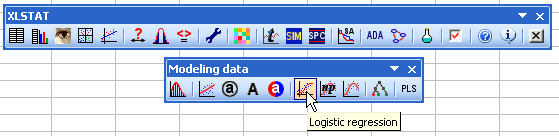When you click on the button, the Logistic regression dialog box appears.

To activate the multinomial logit model, change the response type and choose multinomial. A new box appears where you can choose the control or reference category (in our case we choose a1=0, meaning we set to 0 the relative effect for the first category).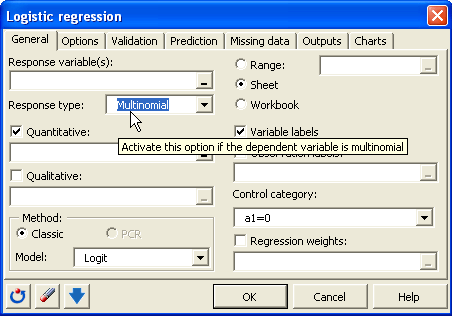Select the data on the Excel sheet. The Response corresponds to the column where the variable to be explained is stored. In this particular case we have two quantitative explanatory variables.

As we selected the column titles of all variables, we have selected the option Variable labels included.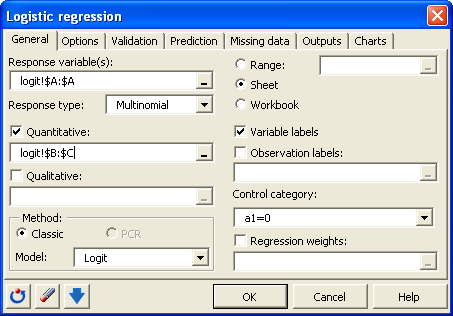Many options are available in the dialog box.

Note: The default options correspond to the basic choice one would make; please consult the XLSTAT help for more details.

The computations begin once you have clicked on the OK button.

## Interpret the results of a multinomial logit model

The following table gives several indicators on the model quality (or goodness of fit). These results are equivalent to the R2 and to the analysis of variance table in linear regression and ANOVA. The most important value to look at is the probability of the Chi-square test on the log ratio. This is equivalent to the Fisher's F test: we try to evaluate if the variables bring significant information by comparing the model as it is defined, with a simpler model with only one constant. In this case, as the probability is lower than 0.0001, we can conclude that significant information is brought by the variables.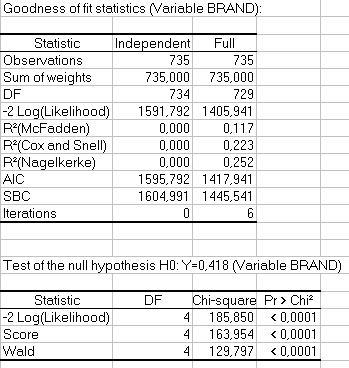The next table gives details on the model. This table is helpful in understanding the effect of the various variables on the categories of the response variable. It is quite different from the logistic regression table. Parameters are obtained for each variable and for each category of the response variable (except the reference category). Odds ratios are also available for a better understanding of the results.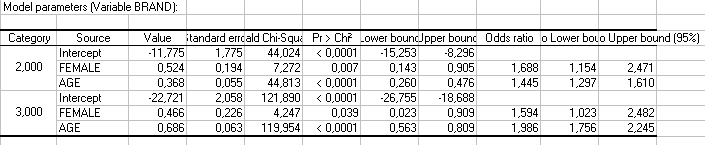Parameters interpretation is not immediate. The model equation for modality 2 is:

Log(P(Response=2)/P(Response=1))=-11.775+0.524*FEMALE+0.368*AGE

For example, we can say that for one unit change in the variable AGE, the log of the ratio of the two probabilities, P(Response=2)/P(Response=1), will be increased by 0.368. Therefore, we can say that, in general, the older a person is, the more he will prefer brand 2. The ratio of the probability of choosing one outcome category over the probability of choosing the reference category is often referred as odds ratios (and it is also sometimes referred as relative risks). So another way of interpreting the regression results is in terms of odds ratios. We can say that for one unit change in the variable AGE, we expect the relative risk of choosing brand 2 over 1 to increase by 1.445.

By looking at the probability of the Chi-squares, we see that the variable that most influences the response variable for both category 2 and 3 is the age of the customer. The intercepts are significant. The marketing experts should focus on older people if they want to increase the market share of the brand 1.

#### Contact our technical support team: support@xlstat.com

https://cdn.desk.com/
false
desk